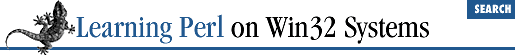home | O'Reilly's CD bookshelfs | FreeBSD | Linux | Cisco | Cisco Exam

### 8.5 Private Variables in Functions

We've already talked about the ``` @_``` variable and how a local copy gets created for each subroutine invoked with parameters. You can create your own scalar, array, and hash variables that work the same way. You do this with the ``` my``` operator, which takes a list of variable names and creates local versions of them (or instantiations , if you like bigger words). Here's that ``` add``` function again, this time using ``` my``` :

```sub add {
my \$sum;         # make \$sum a local variable
\$sum = 0;        # initialize the sum
foreach \$_ (@_) {
\$sum += \$_;  # add each element
}
return \$sum;     # last expression evaluated:
# the sum of elements
}```

When the first body statement is executed, any current value of the global variable ``` \$sum``` is saved away, and a brand new variable named ``` \$sum``` is created (with the value ``` undef``` ). When the subroutine exits, Perl discards the local variable and restores the previous (global) value. This method works even if the ``` \$sum``` variable is currently a local variable from another subroutine (a subroutine that invokes this one, or one that invokes one that invokes this one, and so on). Variables can have many nested local versions, although you can access only one at a time.

Here's a way to create a list of all the elements of an array greater than 100:

```sub bigger_than_100 {
my (@result);         # temporary for holding the return value
foreach \$_ (@_) {     # step through the arg list
if (\$_ > 100) {   # is it eligible?
}
}
return @result;           # return the final list
}```

What if we wanted all elements greater than 50, rather than all elements greater than 100? We'd have to edit the program, changing each 100 to 50. But what if we need both? Well, we can replace the 50 or 100 with a variable reference instead. This change makes the program look like:

```sub bigger_than {
my(\$n,@values);           # create some local variables
(\$n,@values) = @_;        # split args into limit and values
my(@result); # temporary for holding the return value
foreach \$_ (@values) {    # step through the arg list
if (\$_ > \$n) {        # is it eligible?
}
}
@result;                  # return the final list
}
# some invocations:
@new = bigger_than(100,@list);     # @new gets all @list > 100
@this = bigger_than(5,1,5,15,30);  # @this gets (15,30)```

Notice that this time, we used two additional local variables to give names to arguments. This method is fairly common in practice - you can more easily talk about ``` \$n``` and ``` @values``` than talk about ``` \$_``` and ``` @_[1..\$#_]``` , and ``` \$n``` and ``` @values``` are safer as well.

The result of ``` my``` is an assignable list, meaning that it can be used on the left side of an array assignment operator. You can give this list initial values for each of the newly created variables. (If you don't give values to the list, the new variables start with a value of ``` undef``` , just like any other new variable.) As a result, we can combine the first two statements of this subroutine, replacing:

```my(\$n,@values);
(\$n,@values) = @_; # split args into limit and values```

with:

`my(\$n,@values)= @_;`

This is, in fact, a very common Perl-ish thing to do. Local nonargument variables can be given literal values in the same way, such as:

`my(\$sum) = 0; # initialize local variable`

Be warned that despite its appearance as a declaration, ``` my``` is really an executable operator. Good Perl hacking strategy suggests that you bunch all of your ``` my``` operators at the beginning of the subroutine definition, before you get into the meat of the routine.8.4 Arguments8.6 Semiprivate Variables Using local